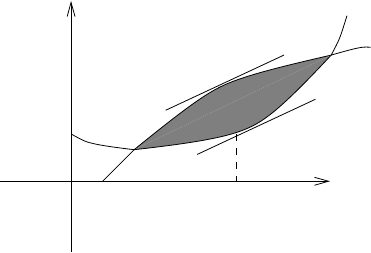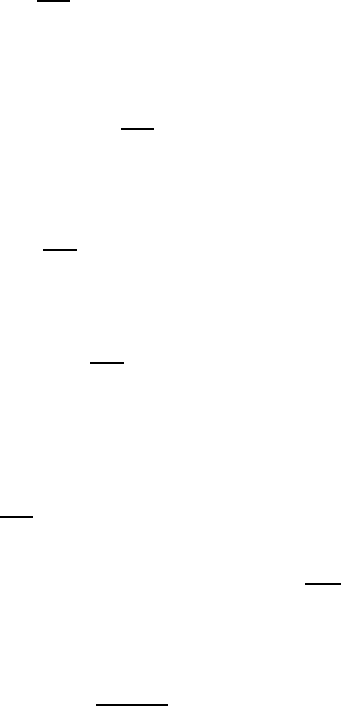Study Guides (380,000)
CA (150,000)
UTSG (10,000)
ANT (300)

# ANT371H1 Study Guide - Unita, Marginal Cost, Simple Algebra

Department
Anthropology
Course Code
ANT371H1
Professor
Holly Wardlow

This preview shows pages 1-2. to view the full 6 pages of the document.3. Proﬁt Under a Monopoly
Under a monopoly a producer has full control over how many units of a product will reach
market. Since market demand and selling price are related by a demand relation, the monopolist
can also control the price at which the commodity sells. Under a free enterprise system it is
assumed that the monopolist will attempt to maximize his or her proﬁt. How many units should
the monopolist produce?
Let R(x) be the revenue associated with the sale of xunits; C(x) the cost to the
monopolist of producing xunits. Then proﬁt is deﬁned to be P(x) , with P(x) = R(x)C(x) .
In Figure 1 typical revenue and cost curves are shown, and the proﬁt zone is shaded in.
0
C
x
y
x0
R(x)
C(x)
Figure 1
Now P0(x) = R0(x)C0(x) , and P0(x) = 0 if and only if R0(x) = C0(x) . So as long as
P00(x)<0 , the proﬁt will be maximized at the production level for which marginal revenue
equals marginal cost. In Figure 1 this optimal production level is denoted by x0; it is the point
where the tangents to the revenue and cost curves are parallel.
Note that the values of price and volume for optimal proﬁt do not depend on the overhead
cost, but if the overhead cost is too high no positive proﬁt may be possible for any realistic
price.
This can be seen in Figure 1 where we have called the overhead cost C0. Increasing C0
may eventually place the entire cost curve above the revenue curve so that there would be no
proﬁtable region.
Example 1. A publisher estimates the cost of producing and selling xcopies of a new science
ﬁction book will be, in dollars,
C(x) = 10,000 + 5x .
1

Only pages 1-2 are available for preview. Some parts have been intentionally blurred.The demand of the book at price pdollars is
x= 4,000 200p .
Find the most proﬁtable sale price.
Solution. Solving the demand relation for p, we ﬁnd
p= 20 x
200 dollars .
Hence total revenue is
R(x) = 20xx2
200
and proﬁt is
P(x) = 20xx2
200 5x10,000 .
For maximum value,
P0(x) = 15 x
100 = 0
x= 1500 .
Observe that
P00(x) = 1
100 <0 for all x ,
so that a true maximum value has been found. The price is then p= 201500
200 = 12.50 (dollars)
and the maximum proﬁt is
P(1500) = 15(1500) (1500)2
200 10,000
= 1,250 (dollars) .
Example 2. A Case Study of Taxation on a Monopoly
In this example we shall construct a simple model to analyse taxation on a monopoly. We
assume that the cost and demand curves for a certain product are both linear, and that a tax
of tdollars per unit is levied on the producer for every unit produced.
2